# An introduction to Flowcharts

What is a Flowchart?
Flowchart is a graphical representation of an algorithm. Programmers often use it as a program-planning tool to solve a problem. It makes use of symbols which are connected among them to indicate the flow of information and processing.
The process of drawing a flowchart for an algorithm is known as “flowcharting”.

Basic Symbols used in Flowchart Designs

1. Terminal: The oval symbol indicates Start, Stop and Halt in a program’s logic flow. A pause/halt is generally used in a program logic under some error conditions. Terminal is the first and last symbols in the flowchart.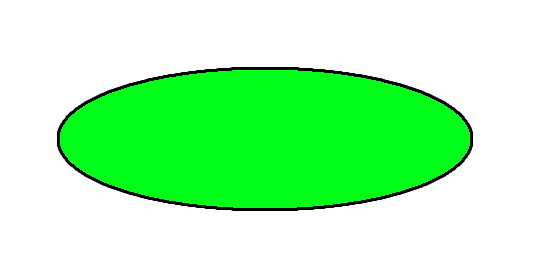• Input/Output: A parallelogram denotes any function of input/output type. Program instructions that take input from input devices and display output on output devices are indicated with parallelogram in a flowchart.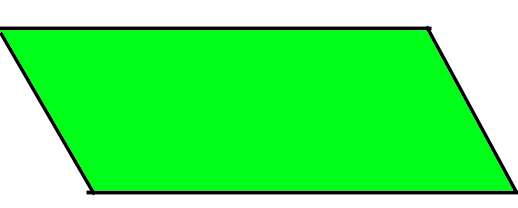• Processing: A box represents arithmetic instructions. All arithmetic processes such as adding, subtracting, multiplication and division are indicated by action or process symbol.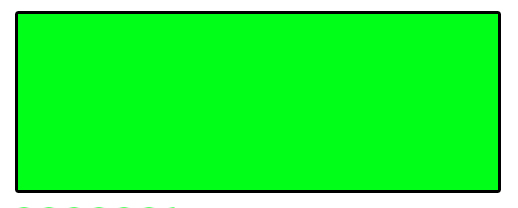• Decision Diamond symbol represents a decision point. Decision based operations such as yes/no question or true/false are indicated by diamond in flowchart.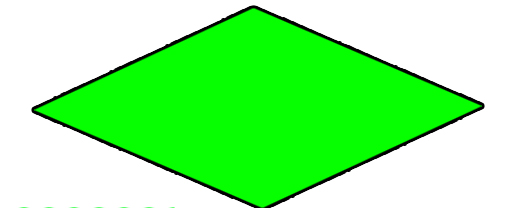• Connectors: Whenever flowchart becomes complex or it spreads over more than one page, it is useful to use connectors to avoid any confusions. It is represented by a circle.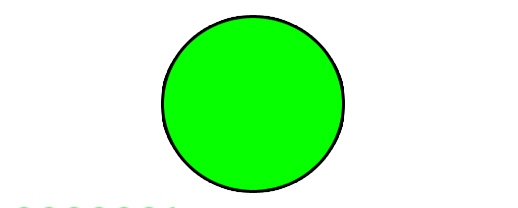• Flow lines: Flow lines indicate the exact sequence in which instructions are executed. Arrows represent the direction of flow of control and relationship among different symbols of flowchart.

• Flowcharts are better way of communicating the logic of system.
• Flowcharts act as a guide for blueprint during program designed.
• Flowcharts helps in debugging process.
• With the help of flowcharts programs can be easily analyzed.
• It provides better documentation.
• Flowcharts serve as a good proper documentation.

• It is difficult to draw flowchart for large and complex programs.
• In this their is no standard to determine the amount of detail.
• Difficult to reproduce the flowcharts.
• It is very difficult to modify the Flowchart.

Example : Draw a flowchart to input two numbers from user and display the largest of two numbers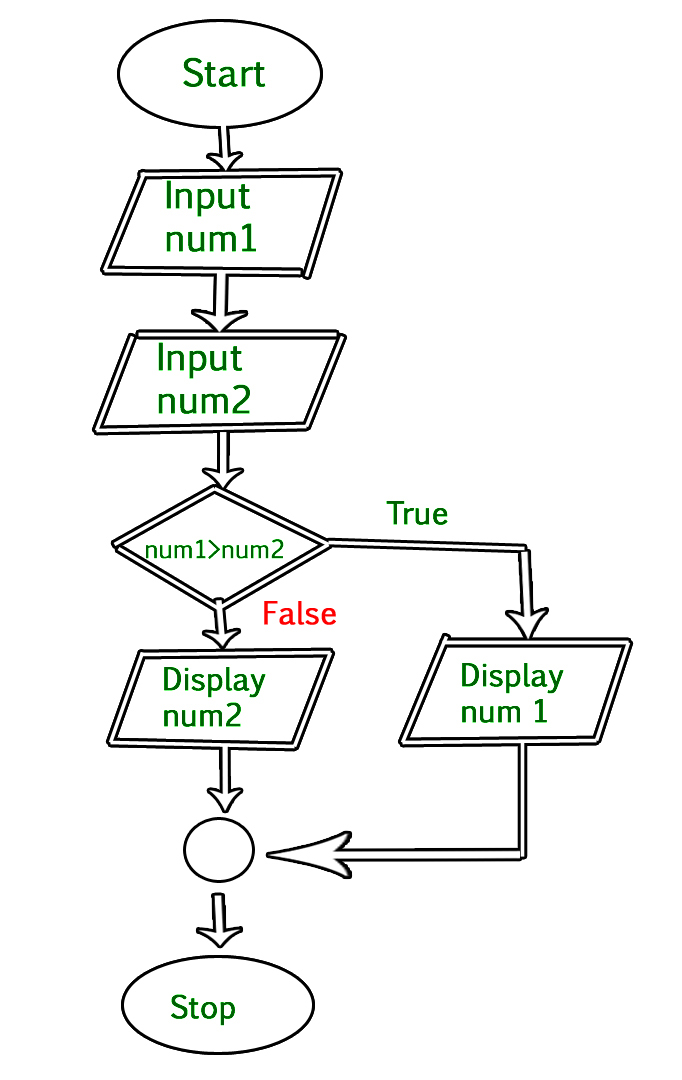## C

 `// C program to find largest of two numbers`   `#include `   `int` `main()` `{` `    ``int` `num1, num2, largest;`   `    ``/*Input two numbers*/` `    ``printf``(``"Enter two numbers:\n"``);` `    ``scanf``(``"%d%d"``, &num1, &num2);`   `    ``/*check if a is greater than b*/` `    ``if` `(num1 > num2)` `        ``largest = num1;` `    ``else` `        ``largest = num2;`   `    ``/*Print the largest number*/` `    ``printf``(``"%d"``, largest);`   `    ``return` `0;` `}`

## C++

 `// C++ program to find largest of two numbers` `#include ` `using` `namespace` `std;` `int` `main()` `{` `    ``int` `num1, num2, largest;`   `    ``/*Input two numbers*/` `    ``cout << ``"Enter two numbers:\n"``;` `    ``cin >> num1;` `    ``cin >> num2;`   `    ``/*check if a is greater than b*/` `    ``if` `(num1 > num2)` `        ``largest = num1;` `    ``else` `        ``largest = num2;`   `    ``/*Print the largest number*/` `    ``cout << largest;`   `    ``return` `0;` `}`

## Java

 `// Java program to find largest of two numbers` `import` `java.util.Scanner;` `public` `class` `largest {` `    ``public` `static` `void` `main(String args[])` `    ``{` `        ``int` `num1, num2, max;`   `        ``/*Input two numbers*/` `        ``Scanner sc = ``new` `Scanner(System.in);` `        ``System.out.println(``"Enter two numbers:"``);`   `        ``num1 = sc.nextInt();` `        ``num2 = sc.nextInt();`   `        ``/*check whether a is greater than b or not*/` `        ``if` `(num1 > num2)` `            ``max = num1;` `        ``else` `            ``max = num2;`   `        ``/*Print the largest number*/` `        ``System.out.println(max);` `    ``}` `}`

## C#

 `// C# program to find largest of two numbers` `using` `System;` `using` `System.IO;`   `class` `GFG` `{` `    ``static` `public` `void` `Main ()` `    ``{` `        `  `        ``int` `num1, num2, max;`   `        ``/*Input two numbers*/` `        ``Console.WriteLine(``"Enter two numbers:"``);`   `        ``num1 = Convert.ToInt32(Console.ReadLine()); ` `        ``num2 = Convert.ToInt32(Console.ReadLine()); `   `        ``/*check whether a is greater than b or not*/` `        ``if` `(num1 > num2)` `            ``max = num1;` `        ``else` `            ``max = num2;`   `        ``/*Print the largest number*/` `        ``Console.WriteLine(max);` `    ``}` `}`   `// This code is contributed by NamrataSrivastava1`

Output

```Enter two numbers:
10 30
30```

References:
Computer Fundamentals by Pradeep K. Sinha and Priti SinhaMy Personal Notes arrow_drop_upCheck out this Author's contributed articles.

If you like GeeksforGeeks and would like to contribute, you can also write an article using contribute.geeksforgeeks.org or mail your article to contribute@geeksforgeeks.org. See your article appearing on the GeeksforGeeks main page and help other Geeks.

Please Improve this article if you find anything incorrect by clicking on the "Improve Article" button below.